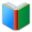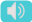# 8th Grade Word List: Math

Words for students of 8th Grade, the page is for math words (List 1), which are essential vocabulary for 8th Grade academic classes.
 Free Online Vocabulary TestK12, SAT, GRE, IELTS, TOEFL
 Actions upon current list

 All lists of current grdae
Spelling Words:

Arts Words:

Social Words:

Math Words:

Science Words:

Literature Words:Animal FarmThe Hound of the Baskervilles
 adjacent anglesare angles that have a common ray coming out of the vertex going between two other rays, with no overlap of the regions
 coefficienta constant number that serves as a measure of some property or characteristic
 constantinvariable; repeating; continually occurring; persistent
 distance formulaalgebraic expression that gives the distance between two points in a particular coordinate system in a particular number of dimensions
 domainfield; territory over which rule or control is exercised; networked computers that share a common address
 formulaplan; directions for making something; a group of symbols that make a mathematical statement
 hypotenusethe side of a right triangle opposite the right angle
 lateral areathe sum of the areas of the lateral (vertical) faces of a cylinder, cone, frustum, or the like
 linear equationa polynomial equation of the first degree
 linear inequalityan inequality which involves a linear function.
 Pythagorean theorema relation in Euclidean geometry among the three sides of a right triangle, it states that the square of the hypotenuse is equal to the sum of the squares of the other two sides
 range of a functionthe set of values of the dependent variable for which a function is defined
 scatter plota type of display using Cartesian coordinates to display values for two variables for a set of data
 scientific notationa method of writing or displaying numbers in terms of a decimal number between 1 and 10 multiplied by a power of 10
 slopebe at an angle; incline; gradient
 slope-intercept formthe equation of a straight line in the form y = mx b where m is the slope of the line and b is its y-intercept
 solidsgeometric figure having three dimensions
 surface areathe extent of a 2-dimensional surface enclosed within a boundary
 termlimited period of time; point in time at which something ends; termination; deadline
 Quick Access Worksheet:
-->